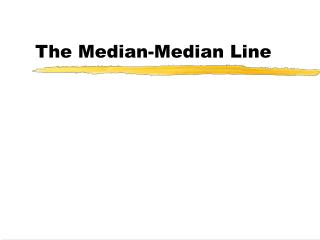Download PresentationThe Median-Median Line

# The Median-Median Line - PowerPoint PPT PresentationDownload Presentation## The Median-Median Line

- - - - - - - - - - - - - - - - - - - - - - - - - - - E N D - - - - - - - - - - - - - - - - - - - - - - - - - - -
##### Presentation Transcript

1. The Median-Median Line

2. Process • Sort the collected data by the independent variable. • Divide the sorted data into three groups. • Find the median x and the median y of each group. • Find the equation of the line that passes through (x1, y1) and (x3, y3).

3. Process • Find the equation of the line parallel to the previous line that passes through (x2, y2). • Find the weighted average of the y-intercepts. b=(b1+b2+b1)/3 • The median-median line is the line parallel to the previous two lines with the weighted y-intercept.

4. Wave Data

5. Process • Sort the collected data by the independent variable. • Divide the sorted data into three groups. • Find the median x and the median y of each group. • Find the equation of the line that passes through (x1, y1) and (x3, y3).

6. Process • Find the equation of the line parallel to the previous line that passes through (x2, y2). • Find the weighted average of the y-intercepts. b=(b1+b2+b1)/3 • The median-median line is the line parallel to the previous two lines with the weighted y-intercept.